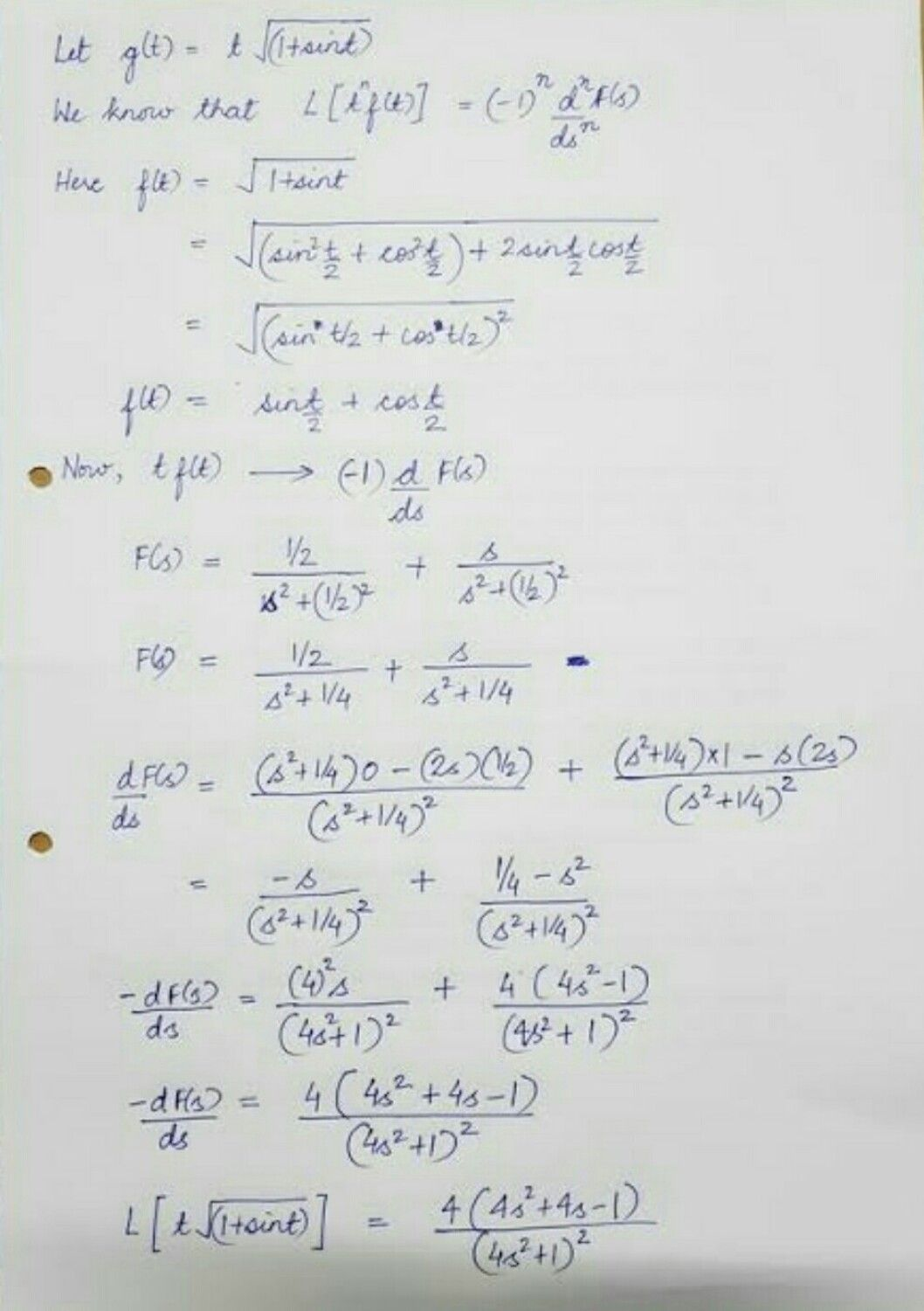Symbol
Problem$4$ Find the Laplace Transform of the periodic function f whose graph is given below. $1$ $1$ $2α$ $4a$ $\bar{6a}$ $-$ $1$
Calculus
Search count: 106
SolutionQanda teacher - Priyathank you